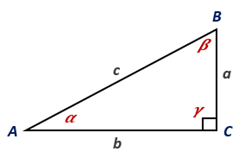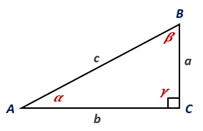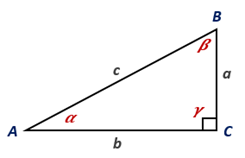# For the right triangle shown, express each of the following in terms of a, b, and c: a) sin α b) tan β### Elementary Geometry For College St...

7th Edition
Alexander + 2 others
Publisher: Cengage,
ISBN: 9781337614085### Elementary Geometry For College St...

7th Edition
Alexander + 2 others
Publisher: Cengage,
ISBN: 9781337614085

#### Solutions

Chapter
Section
Chapter 11.CT, Problem 1CT
Textbook Problem

## For the right triangle shown, express each of the following in terms of a, b, and c: a) sin αb) tan βExpert Solution
To determine

a

To express:

The ratio sin α in terms of a, b, and c.

Solution:

The value of sin α in terms of a, b, and c is sin α = ac.

### Explanation of Solution

Definition:

In a right triangle, the sine ratio for an acute angle is the ratio oppsite sidehypotenuse.

Given:

The right triangle shown belowFrom the figure given,

For the angle α, opposite side = a and hypotenuse = c.

By the definition, sin α = oppsite sidehypotenuse

sin α = ac

Conclusion:

The value of sin α in terms of a, b, and c is sin α = ac.

Expert Solution
To determine

b.

To express:

The given tan β in terms of a, b, and c.

Solution:

The value of tan β in terms of a, b, and c is tan β = ba.

### Explanation of Solution

Definition:

In a right triangle, the tangent ratio for an acute angle is the ratio oppsite sideadjacent side.

Given:

The right triangle shown belowCalculation:

For the angle β, opposite side = b and adjacent side = a.

By the definition, tan β= oppsite sideadjacent side

tan β = ba

Conclusion:

The value of tan β in terms of a, b, and c is tan β =ba.

### Want to see more full solutions like this?

Subscribe now to access step-by-step solutions to millions of textbook problems written by subject matter experts!

Get Solutions

### Want to see more full solutions like this?

Subscribe now to access step-by-step solutions to millions of textbook problems written by subject matter experts!

Get Solutions